Question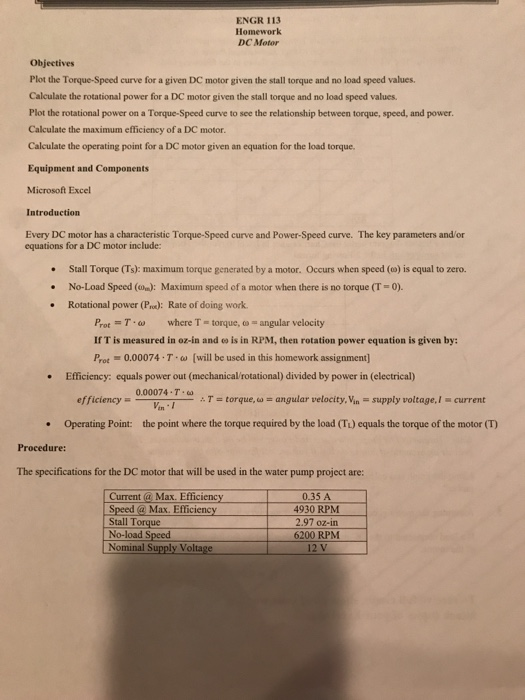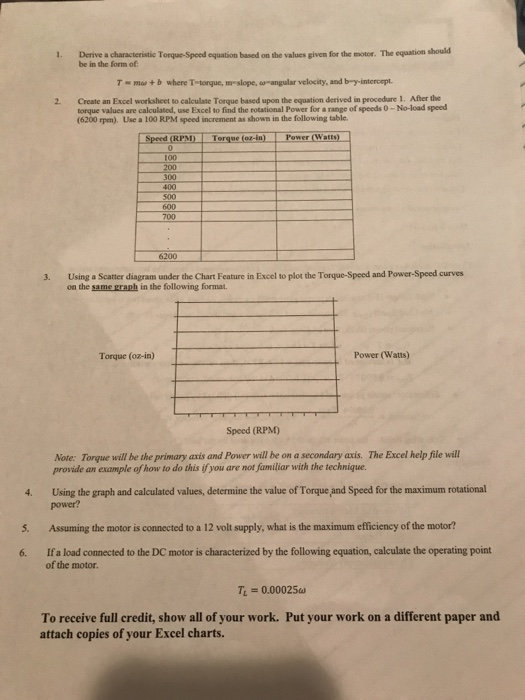need the answer for the question no. 1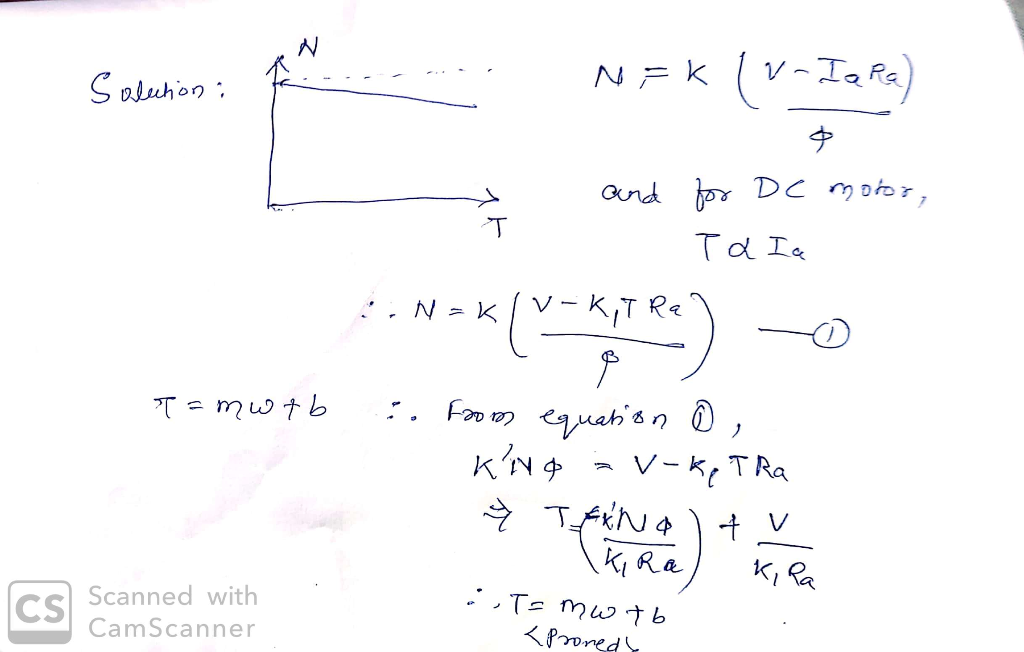Question 1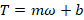Given that the Stall Torque is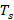. This is the maximum torque generated by the motor. This occurs when the speed is zero.

So when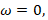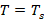Substituting this, we get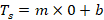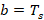Now the no load speed is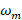. This is the speed when there is no torque.

So when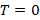,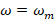Substituting this we get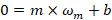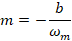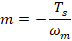Hence we can write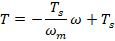So the characteristic Torque – Speed equation isIn the specification, we can see that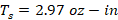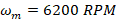So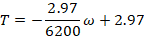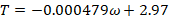Question (2)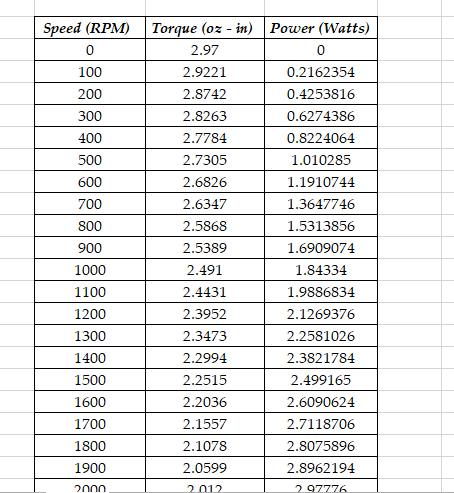#### Earn Coins

Coins can be redeemed for fabulous gifts.

Similar Homework Help Questions
• ### Question 3: DC motors can be simplistically modeled as shown in Figure 5 MoTor back emf Vi: +1 Re...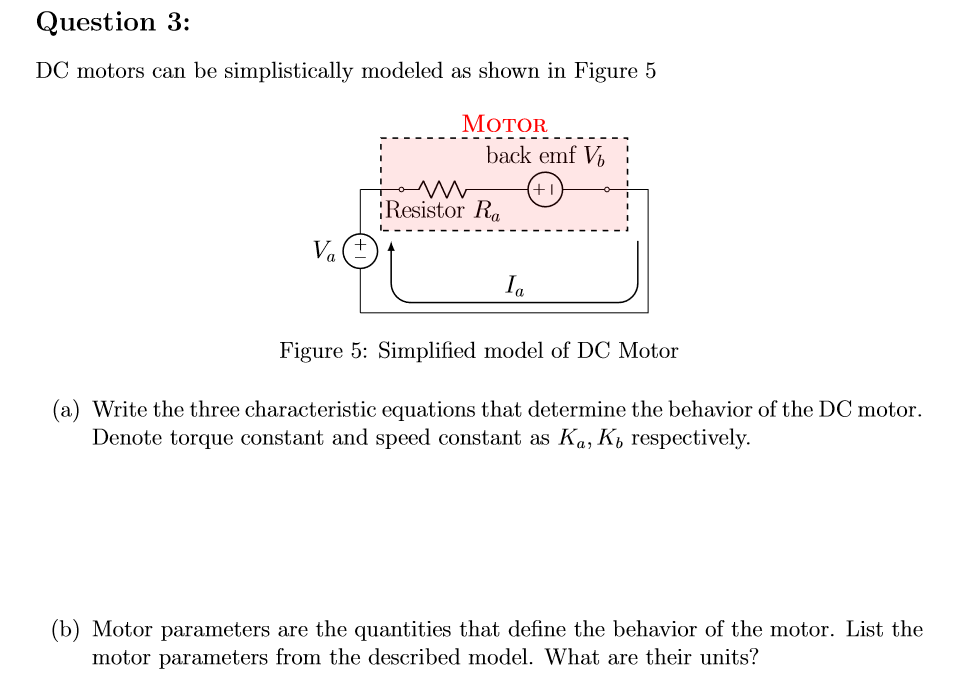Question 3: DC motors can be simplistically modeled as shown in Figure 5 MoTor back emf Vi: +1 Resistor Ra Ia Figure 5: Simplified model of DC Motor (a) Write the three characteristic equations that determine the behavior of the DC motor. Denote torque constant and speed constant as Ka, K, respectively. (b) Motor parameters are the quantities that define the behavior of the motor. List the motor parameters from the described model. What are their units? (c) For given...

• ### A 12-pole, brushless, DC motor has a no-load speed of 5000rpm and a stall torque of 50 in-oz when operating at rated voltage of 60V. Testing shows that no-load speed and stall torque vary linearly wi...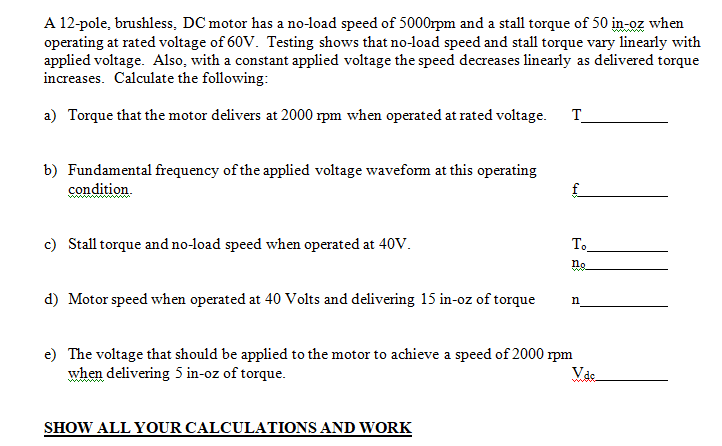A 12-pole, brushless, DC motor has a no-load speed of 5000rpm and a stall torque of 50 in-oz when operating at rated voltage of 60V. Testing shows that no-load speed and stall torque vary linearly with applied voltage. Also, with a constant applied voltage the speed decreases linearly as delivered torque increases. Calculate the following: Torque that the motor delivers at 2000 rpm when operated at rated voltage. T a) b) Fundamental frequency of the applied voltage waveform at this...

• ### QUESTIONS Question-3: Draw "speed vs. torque" curve using data in Table-1. The completed graph represents the speed vs. torque characteristics of a typical DC shunt-wound motor. Terax Qu...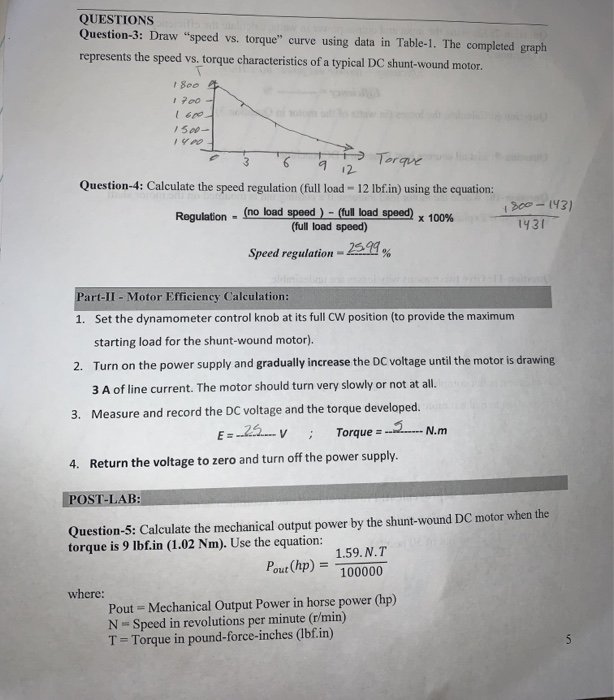QUESTIONS Question-3: Draw "speed vs. torque" curve using data in Table-1. The completed graph represents the speed vs. torque characteristics of a typical DC shunt-wound motor. Terax Question-4: Calculate the speed regulation (full load - 12 lbf.in) using the equation: 2-143) 143 (no load Regulation (full load speed) Speed regulation- % Part-II - Motor Efficiency Calculation: Set the dynamometer control knob at its full CW position (to provide the maximum starting load for the shunt-wound motor). Turn on the power...

• ### Q1b) DC Machines [ 6 marks ] You are given a 24 V DC motor. When...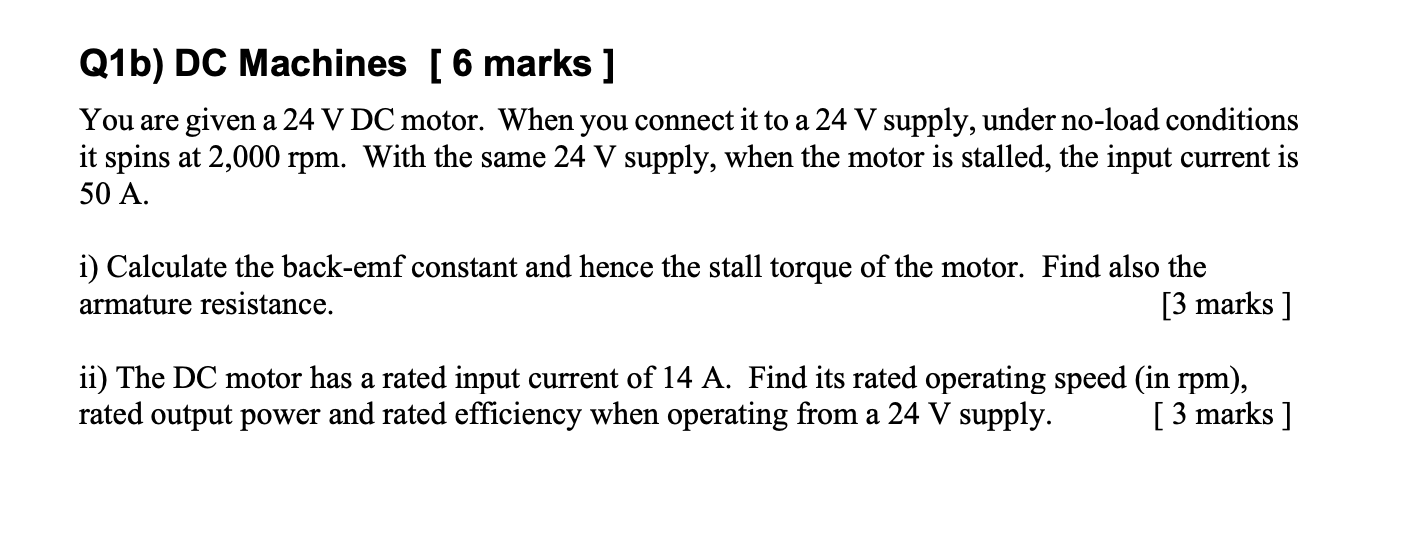Q1b) DC Machines [ 6 marks ] You are given a 24 V DC motor. When you connect it to a 24 V supply, under no-load conditions it spins at 2,000 rpm. With the same 24 V supply, when the motor is stalled, the input current is 50 A. i) Calculate the back-emf constant and hence the stall torque of the motor. Find also the armature resistance. [3 marks ] ii) The DC motor has a rated input current of...

• ### A DC motor, when supplied from a 211 V power supply is observed to rotate under...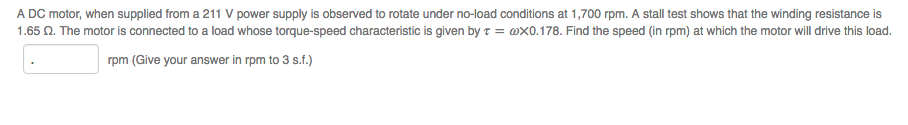A DC motor, when supplied from a 211 V power supply is observed to rotate under no-load conditions at 1,700 rpm. A stall test shows that the winding resistance is 1.65 ? The motor is connected to a load whose torque-speed characteristic is given by ? = ?×0.178. Find the speed (in rpm) at which the motor will drive this load. rpm (Give your answer in rpm to 3 s.f.)

• ### If a manufacturer's specifications for a PM DC motor are as follows, what are the motor's...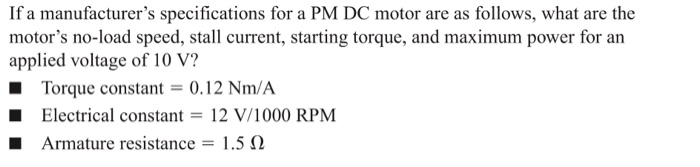If a manufacturer's specifications for a PM DC motor are as follows, what are the motor's no-load speed, stall current, starting torque, and maximum power for an applied voltage of 10 V? ■ Torque constant 0.12 Nm/A Electrical constant 12 V/1000 RPM Armature resistance-: 1.5 Ω

• ### For a PMDC motor powered by a 12 V DC source, the no-load speed and stall...

For a PMDC motor powered by a 12 V DC source, the no-load speed and stall torque are 3800 RPM and 30 mNm, respectively. If the motor is running at 2500 RPM, find a) Shaft torque (Tshaft), b) Rotor current (Im), c) Back emf (Eb), d) Electrical power consumed, e) Shaft power, and f) Power loss in rotor coil.

• ### A DC permanent magnet motor draws 5A from a 200V DC source, under no-load conditions, and...

A DC permanent magnet motor draws 5A from a 200V DC source, under no-load conditions, and draws 50A from the same source, and has a speed of 2500rpm at full load. Ra = 1.4Ω. Determine: a) the rotation loss* (W) b) the no-load speed (rpm) c) the output torque (Nm) d) the efficiency of the motor at full load. (*Assume the rotational loss is proportional to speed!)

• ### DC series MOTOR... LAB I need help answering question 6 and 7. Results and Discussion: 1. Plot machine speed vs resistance 2. Plot machine speed vs current 3. Explain the above plots 4. Using DC M...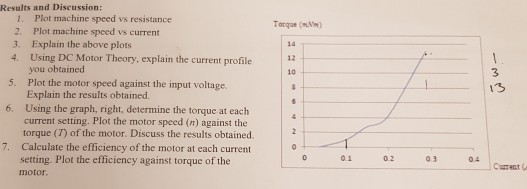DC series MOTOR... LAB I need help answering question 6 and 7. Results and Discussion: 1. Plot machine speed vs resistance 2. Plot machine speed vs current 3. Explain the above plots 4. Using DC Motor Theory, explain the current profile Totque (mV) 12 you obtained Plot the motor speed against the input voltage. Explain the results obtained. Using the graph, right, determine the torque at each current setting. Plot the motor speed (n) against the torque (T) of the...

• ### The permanent DC motor model CDP3450 by ABB is rated at 1 Hp, 2 500 RMP...

The permanent DC motor model CDP3450 by ABB is rated at 1 Hp, 2 500 RMP at 90v (no load). The armature resistance is 0.425 Ohm. a. The continuous current rating of this motor is 9.7 Amp, what is the maximum continuous torque rating? b. The motor is to drive a load of 2NM torque while maintaining a constant speed of 2000 RPM. What is the required supply current and voltage? c. With 90v armature voltage, what is the maximum...